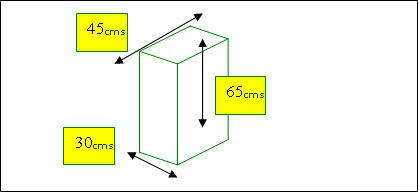All freight transportation modes are governed by weight and cubic measurement factors When you send your goods by Airfreight however the Carrier will charge on the Actual Weight or the Volumetric Weightc, whichever is deemed to be the greater. The higher weight is then called the "Chargeable Weight"

The IATA / Airline Cubic Allowance Factor for Air Freight Shipments is
1 Kilo = 6,000 Cubic Centimetres.

The illustration below shows you how to calculate the Volumetric Chargeable Weight as follows :

• Let’s assume the actual gross weight of this carton is 14 Kilos.
• The carton measures 30 × 45 × 65cms which is 87750 Cubic Centimetres.
• Divide this figure by the IATA/Airline Cubic Allowance Factor of 6000 Cubic Centimetres = 14.625 Kilos.
• In this instance the Air Freight charge will be calculated on 15 Kilos volumetric, rather than the 14 kilos actual weight.